Courses

# Shear, Torsion, Bond, Anchorage & Development Length Civil Engineering (CE) Notes | EduRev

## Topic wise GATE Past Year Papers for Civil Engineering

Created by: Gate Gurus

## Civil Engineering (CE) : Shear, Torsion, Bond, Anchorage & Development Length Civil Engineering (CE) Notes | EduRev

The document Shear, Torsion, Bond, Anchorage & Development Length Civil Engineering (CE) Notes | EduRev is a part of the Civil Engineering (CE) Course Topic wise GATE Past Year Papers for Civil Engineering.
All you need of Civil Engineering (CE) at this link: Civil Engineering (CE)

Q.1  An RCC beam of rectangular cross-section has factored shear of 200 kN at its critical section. Its width b is 250 mm and effective depth d is 350 mm. Assume design shear strength τc of concrete as 0.62 N/mm2 and maximum allowable shear stress τc max in concrete as 2.8 N/mm2. If two legged 10 mm diameter vertical stirrups of Fe250 grade steel are used, then the required spacing (in cm, up to one decimal place) as per limit state method will be ________    [2018 : 2 Marks, Set-I]
Solution:

Nominal shear stress,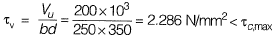SF taken by stirrups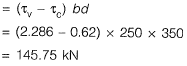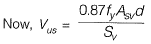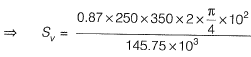= 82 mm = 8.2 cm

Q.2  As per IS 456 : 2000 for the design of reinforced concrete beam, the maximum allowable shear stress (τcmax) depends on the    [2016 : 1 Mark, Set-II]
(d) grade of concrete and percentage of reinforcemen
Ans.
(B)
Solution:
From table 20 of IS 456 : 2000 it is evident that τcmax values are dependent only on concrete grade.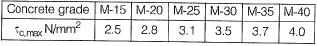Q.3 In shear design of an RC beam, other than allowable shear strength of concrete (τc), there is also an additional check suggested in IS 456 : 2000 with respect to the maximum permissible shear stress (τcmax). The check for (τcmax) is required to take care of    [2016 : 1 Mark, Set-I]
(a) additional shear resistance from reinforcing steel
(c) possibility of failure of concrete by diagonal tension
(d) possibility of crushing of concrete by diagonal compression
Ans.
(D)
Solution:
The check for τcmax is an additional check to take care of possibility of crushing of concrete by design of compression.

Q.4 The development length of a deformed reinforcement bar can be expressed as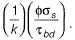From the  IS: 456-2000 , the value of k can be calculated as ________.    [2015 : 1 Mark, Set-I]
Solution:

Bond strength of concrete
= Tensile force in steel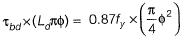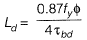For deformed bars τbd value is increased by 60%,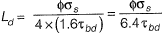∴ k = 6.4

Q.5 A rectangular beam of width (b) 230mm and effective depth (d) 450 mm is reinforced with four bars of 12 mm diameter. The grade of concrete is M20 and grade of steel is Fe500. Given that for M20 grade of concrete the ultimate shear strength, τuc = 0.36 N/mm2 for steel percentage, p = 0.25, and τuc= 0.48 N/mm2 for p = 0.50. For a factored shear force of 45 kN, the diameter (in mm) of Fe500 steel two legged stirrups to be used at spacing of 375 mm, should be    [2014 : 2 Marks, Set-I]
(a) 8
(b) 10
(c) 12
(d) 16
Ans.
(A)
Solution: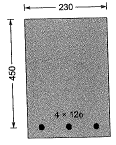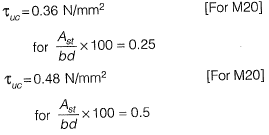Factored SF = 45 kN - Vu
We have to calculate the dia of Fe 500 2-Legged stirrup to be used at a spacing of 325 mm c/c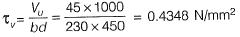∴ %tensile steel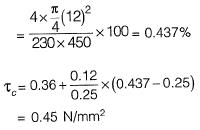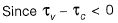⇒ Min shear reinforcement is required
⇒ Min shear reinforcement is given by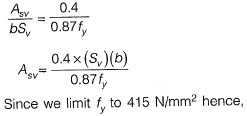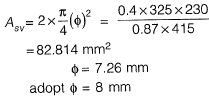Q.6 As per IS 456 : 2000 for M20 grade concrete and plain bars in tension the design bond stress τbd = 1.2 MPa. Further, IS 456 : 2000 permits this design bond stress value to be increased by 60% for HYSD bars. The stress in the HYSD reinforcing steel bars in tension, σs = 360 MPa. Find the required development length, Ld, for HYSD bars in terms of the bar diameter, φ.______    [2013 : 1 Mark]
Solution: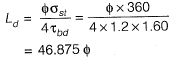Q.7 Consider a bar of diameter ‘D embedded in a large concrete block as shown in the adjoining figure, with a pull out force P being applied. Let σb and σst be the bond strength (between the bar and concrete) and the tensile strength of the bar, respectively. If the block is held in position and it is assumed that the material of the block does not fail, which of the following options represents the maximum value of P?    [2011 : 2 Marks]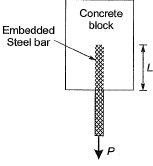(a) Maximum of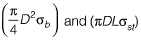(b) Maximum of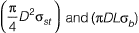(c) Minimum of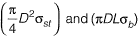(d) Minimum of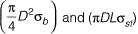Ans.
(C)
Solution: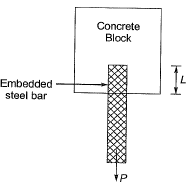Maximum force resisted by bond strength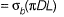Maximum tensile force resisted by bar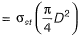∴ Maximum value of P = Minimum of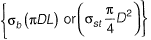Q.8 Consider two RCC beams, Pand Q, each having the section 400 mm x 750 mm (effective depth, d = 750 mm) made with concrete having τcmax = 2.1 N/mm2. For the reinforcement provided and the grade of concrete used, it may be assumed that the τc = 0.75 N/mm2. The design shear in beam P is 400 kN and in beam Q is 750 kN. Considering the provisions of IS 456 : 2000, which of the following statements is TRUE?    [2011 : 2 Marks]
(a) Shear reinforcement should be designed for 175 kN for beam Pand the section for beam Q should be revised.
(b) Nominal shear reinforcement is required for beam Pand the shear reinforcement should be designed for 120 kN for beam Q.
(c) Shear reinforcement should be designed for 175 kN for beam Pand the shear reinforcement should be designed for 525 kN for beam Q.
(d) The sections for both beams Pand Q need to be revised.
Ans.
(A)
Solution:
Shear strength of the concrete = τc  x Bd
= 0.75 x 400 x 750 x 10-3 = 225 kN
Maximum shear strength of concrete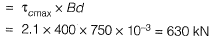Design shear in beam P is 400 kN which is less than the maximum shear that the concrete can sustain. But the design shear is more than the nominal shear of 225 kN.
∴ Shear reinforcement is designed for
= 400-225 = 175 kN
Design shear in beam Q is 750 kN which is more than the maximum shear that the concrete can sustain. Hence the beam Q should be redesigned.

Q.9 Consider a reinforcing bar embedded in concrete. In a marine environment this bar undergoes uniform corrosion, which leads to the deposition of corrosion products on its surface and an increase in the apparent volume of the bar. This subjects the surrounding concrete to expansive pressure As a result , corrosion induced cracks appear at the surface of concrete. Which of the following statements is TRUE?    [2011 ; 1 Mark]
(a) Corrosion causes circumferential tensile stresses in concrete and the cracks will be parallel to the corroded reinforcing bar.
(b) Corrosion causes radial tensile stresses in concrete and the cracks will be parallel to the corroded reinforcing bar.
(c) Corrosion causes circumferential tensile stresses in concrete and the cracks will be perpendicular to the direction of the corroded reinforcing bar.
(d) Corrosion causes radial tensile stresses in concrete and the cracks will be perpendicular to the direction of the corroded reinforcing bar.
Ans.
(A)
Solution: Corrosion in steel occupies more volume than volume of reinforcing steel, hence exerts radial pressure on adjoining concrete layer. As a consequence, cracking results along reinforcing bars and concrete starts spalling.

Offer running on EduRev: Apply code STAYHOME200 to get INR 200 off on our premium plan EduRev Infinity!

69 docs

,

,

,

,

,

,

,

,

,

,

,

,

,

,

,

,

,

,

,

,

,

,

,

,

,

,

,

,

,

,

;